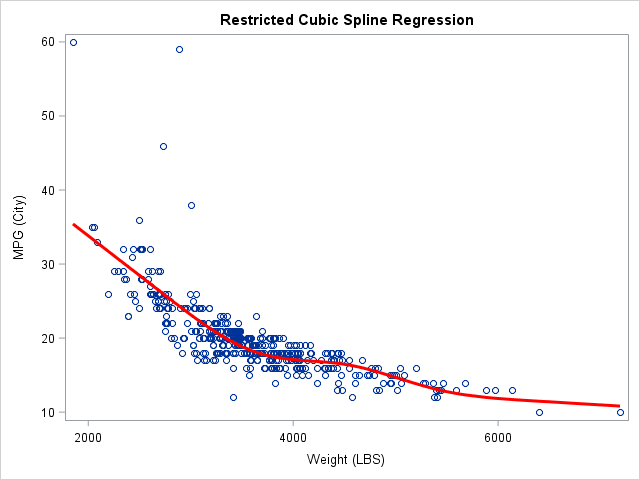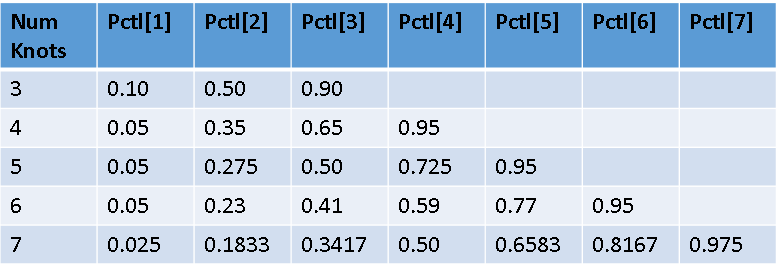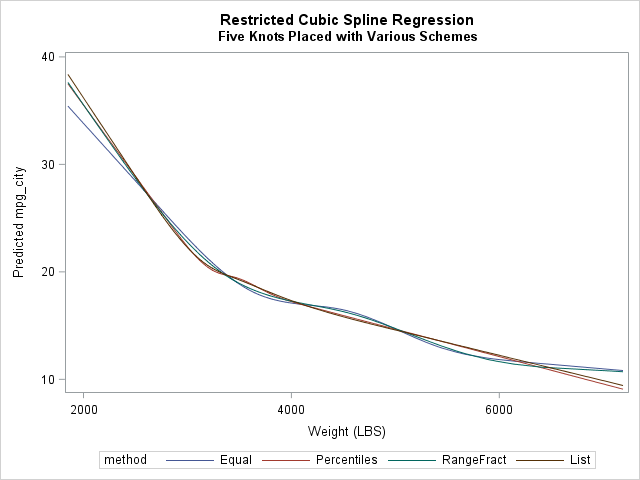Restricted cubic splines are a powerful technique for modeling nonlinear relationships by using linear regression models. I have attended multiple SAS Global Forum presentations that show how to use restricted cubic splines in SAS regression procedures. However, the presenters have all used the %RCSPLINE macro (Frank Harrell, 1988) to generate a SAS data set that contains new variables for the spline basis functions. They then use those basis variables in a SAS regression procedure.

Since SAS 9.3, many SAS regression procedures provide a native implementation of restricted cubic splines by using the EFFECT statement in SAS. This article provides examples of using splines in regression models. Because some older procedures (such as PROC REG) do not support the EFFECT statement, the article also shows how to use SAS procedures to generate the spline basis, just like the %RCSPLINE macro does.

If you are not familiar with splines and knots, read the overview article "Understanding splines in the EFFECT statement." You can also read the documentation for the EFFECT statement, which includes an overview of spline effects as well as a brief description of restricted cubic splines, which are also called "natural cubic splines." I think the fact that the SAS documentation refers to the restricted cubic splines as "natural cubic splines" has prevented some practitioners from realizing that SAS supports restricted cubic splines.

### Regression with restricted cubic splines in SASThis section provides an example of using splines in PROC GLMSELECT to fit a GLM regression model. Because the functionality is contained in the EFFECT statement, the syntax is the same for other procedures. For example, if you have a binary response you can use the EFFECT statement in PROC LOGISTIC. If you a fitting a generalized linear model or a mixed model, you can use PROC GLMMIX.

This example fits the MPG_CITY variable as a function of the WEIGHT variable for vehicles in the Sashelp.Cars data set. The data and a scatter plot smoother are shown in the adjacent graph. (To produce the graph, the following statements sort the data, but that is not required.) The smoother is based on a restricted spline basis with five knots. Notice that the SELECTION=NONE option in the MODEL statement turns off the variable selection features of PROC GLMSELECT, which makes the procedure fit one model just like PROC GLM.

```/* create sample data; sort by independent variable for graphing */ proc sort data=sashelp.cars out=cars(keep=mpg_city weight model); by weight; run;   /* Fit data by using restricted cubic splines. The EFFECT statement is supported by many procedures: GLIMMIX, GLMSELECT, LOGISTIC, PHREG, PLS, QUANTREG, ROBUSTREG, ... */ ods select ANOVA ParameterEstimates SplineKnots; proc glmselect data=cars; effect spl = spline(weight / details naturalcubic basis=tpf(noint) knotmethod=percentiles(5) ); model mpg_city = spl / selection=none; /* fit model by using spline effects */ output out=SplineOut predicted=Fit; /* output predicted values for graphing */ quit;   title "Restricted Cubic Spline Regression"; proc sgplot data=SplineOut noautolegend; scatter x=weight y=mpg_city; series x=weight y=Fit / lineattrs=(thickness=3 color=red); run;```

The EFFECT statement with the SPLINE option is used to generate spline effects from the WEIGHT variable. The effects are assigned the collective name 'spl'. Putting 'spl' on the MODEL statement says "use the spline effects that I created." You can specify multiple EFFECT statements. Spline effects depend on three quantities: the kind of spline, the number of knots, and the placement of the knots.

• You specify restricted cubic splines by using the NATURALCUBIC BASIS=TPF(NOINT) options. Technically you do not need the NOINT suboption, but I recommend it because it makes the parameter estimates table simpler.
• The KNOTMETHOD= option enables you to specify the number and placement of knots. In this example, PERCENTILES(5) places knots at five evenly spaced percentiles of the explanatory variable, which are the 16.6%, 33.3%, 50%, 66.6%, and 83.3% percentiles. Equivalently, the knots are placed at the 1/6, 2/6, 3/6, 4/6, and 5/6 quantiles.

### The number and placement of knots for splines

Most researchers use a small number of knots, often three to five. The exact location of knots is not usually critical: if you change the positions slightly the predicted values of the regression change only slightly. A common scheme is to place the knots at fixed percentiles of the data, as shown above. Alternatively, Harrell (Regression Modeling Strategies, 2010 and 2015) suggests heuristic percentiles as shown below, and this scheme seems to be popular among biostatisticians.The KNOTMETHOD= option on the EFFECT statement provides several options for specifying the locations of knots. Try each of the following options and look at the "SplineKnots" table to see the positions of the knots:

• KNOTMETHOD=PERCENTILES(5): Places knots at the percentiles 1/6, 2/6, ..., 5/6. An example is shown above.
• KNOTMETHOD=EQUAL(5): Places knots evenly within the range of the data. If δ = (max-min)/6, then the knots are located at min + i δ for i=1, 2, ..., 5.
• KNOTMETHOD=RANGEFRACTIONS(0.05 0.275 0.50 0.725 0.95): If you want knots placed unevenly with the range of the data, use this option. For example, the value 0.05 means "place a knot 5% along the data range" and 0.272 means "place a knot 27.5% along the data range." You can separate list values by using spaces or commas.
• KNOTMETHOD=LIST(2513, 3174, 3474.5, 3903, 5000): This option enables you to list the locations (in data coordinates) of the knots. You can use this option to specify Harrell's schemes. For example, Harrel suggests the 5th, 27.5th, 50th, 72.5th, and 95th percentiles when you use five knots. You can use PROC UNIVARIATE to find these percentiles for the WEIGHT variable and then type the results into the KNOTMETHOD=LIST option.The adjacent graph shows the predicted values for the four different knot placement methods. (Click to enlarge.) You can see that the general shape of the regression curves is similar regardless of the placement of knots. The differences can be understood by looking at the placement of the first and last knots. The slope of the natural cubic spline fit is linear past the extreme knots, so the placement of the extreme knots dictate where the predictions become linear. For the EQUAL and RANGEFRACTION methods, the largest knots are placed at WEIGHT=6300 and WEIGHT=6923, respectively. Consequently, the predictions for large values of WEIGHT look more cubic than linear. In contrast, the largest knot for the PERCENTILES method is placed at 4175 and the largest LIST knot is 5000. The predictions past those values are linear.

### Automate Harrell's knot placement suggestions

In the previous section, I manually typed in the values that correspond to the 5th, 27.5th, 50th, 72.5th, and 95th percentiles of the WEIGHT variable, as suggested by Harrell's scheme. However, it is not difficult to automate that step. You can use PROC UNIVARIATE to write the percentile values to a SAS data set and then use a short DATA _NULL_ step to store those values into a macro variable. The macro variable can then be used as the argument to the KNOTMETHOD=LIST option, as follows:

```proc univariate data=cars noprint; var weight; output out=percentiles pctlpts=5 27.5 50 72.5 95 pctlpre=p_; / * specify the percentiles */ run;   data _null_; set percentiles; call symput('pctls',catx(',', of _numeric_)); /* put all values into a comma-separated list */ run;   %put &=pctls; /* optional: display the list of values */ ... effect spl = spline(weight / ... knotmethod=list(&pctls) ); /* use the macro variable here */ ...```
`PCTLS=2513,3174,3474.5,3903,5000`

### Write the spline basis functions to a SAS data set

As mentioned eaerlier, not every SAS procedure supports the EFFECT statement. However, the GLMSELECT, LOGISTIC, and GLIMMIX procedures all provide an OUTDESIGN= option, which enables you to output the design matrix that contains the spline basis functions. Furthermore, PROC LOGISTIC supports the OUTDESIGNONLY option and PROC GLIMMIX supports the NOFIT option so that the procedures do not fit the model but only output the design matrix.

You can use the variables in the design matrix to perform regression or other analyses. For example, the following example writes the restricted cubic basis functions to a data set, then uses PROC REG to fit the model:

```/* create SplineBasis = data set that contains spline basis functions */ proc glmselect data=cars outdesign(addinputvars fullmodel)=SplineBasis; effect spl = spline(weight / naturalcubic basis=tpf(noint) knotmethod=percentiles(5)); model mpg_city = spl / selection=none; quit;   /* use design variables in other procedures */ proc reg data=SplineBasis; model mpg_city = spl_:; output out=out p=p; run;```

One last comment: the basis functions that are generated by the EFFECT statement are not equal to the basis functions created by the %RCSPLINE macro, but they are equivalent. The EFFECT statement uses the definition from The Elements of Statistical Learning (Hastie, Tibshirani, and Friedman, 2009, 2nd Ed, pp. 144-146). The %RCSPLINE macro implements scaled versions of the basis function. Thus parameter estimates will be different but the predicted values will be the same.

### Summary

You can use the NATURALCUBIC BASIS=TPF(NOINT) option in the EFFECT statement in SAS to perform regression with restricted cubic splines, which are also called natural cubic splines. You can use the KNOTMETHOD= option to specify the number and placement of the knots. The EFFECT statement is supported by the GLMSELECT, LOGISTIC, and GLIMMIX procedures, among others. These procedures enable you to output a design matrix that contains the spline basis functions, which you can use in procedures that do not support the EFFECT statement.

This article was inspired by several talks that I heard at SAS Global Forum. For more information on this topic, see the following:

The post Regression with restricted cubic splines in SAS appeared first on The DO Loop.